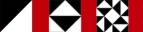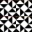Next Higher-Order Parsing 38

# 字句解析Lexing

```        my %builtin = (sin => 1, cos => 1, sqrt => 1);

sub make_tokens {
my \$s = shift;
my @tokens;
my \$lexer = sub {
TOP: {
return undef          if \$s =~ m/\G\z/gxc;
return ["NUMBER", \$1] if \$s =~ m/\G (\d+) /gxc;
return \$builtin{\$1} ? ["FUNCTION", \$1]
: ["VAR", \$1]
if \$s =~ m/\G ([A-Za-z]\w*) /gxc;
return ["^"]          if \$s =~ m/\G (  \^ | \*\*  ) /gxc;
return ["+"]          if \$s =~ m/\G \+ /gxc;
return ["*"]          if \$s =~ m/\G  \*  /gxc;
return ["("]          if \$s =~ m/\G \( /gxc;
return [")"]          if \$s =~ m/\G \) /gxc;
redo TOP              if \$s =~ m/\G \s+ /gxc;
die "Unknown character '\$1' at ..."
if \$s =~ m/\G (.) /gxc;
}};
while (my \$token = \$lexer->()) {
push @tokens, \$token;
}
return @tokens;
}
```
• レクサが^**も認識しながら差を吸収しているところに注目

Notice how the lexer can recognize both ^ and ** and eliminate the distinction

• **が2つの乗算記号ではなくべき乗の演算子として解析されているところにもかな

Also notice how ** is lexed as a power operator, not as two multiplication signs

 NextCopyright © 2007 M. J. Dominus